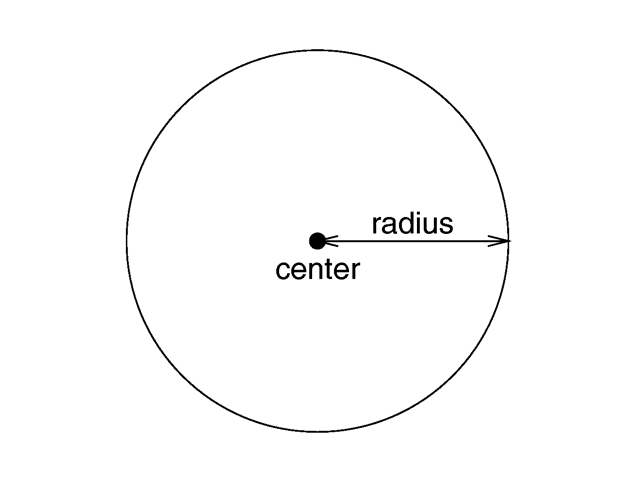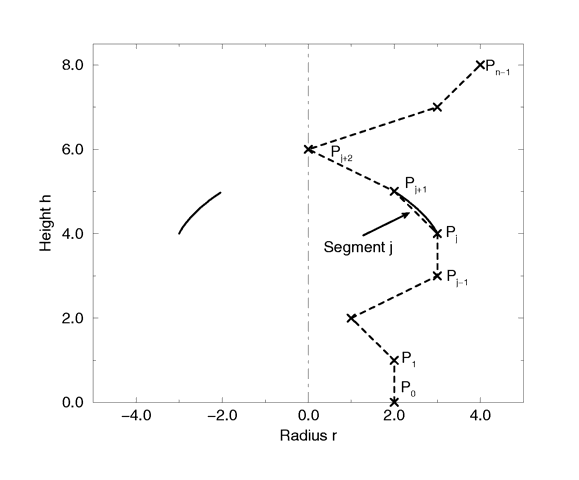### Section 7.5.2.9Sphere

The syntax of the sphere object is:The geometry of a sphere.

Because spheres are highly optimized they make good bounding shapes (if manual bounding seems to be necessary).

The superquadric ellipsoid is an extension of the quadric ellipsoid. It can be used to create boxes and cylinders with round edges and other interesting shapes. Mathematically it is given by the equation:

f(x, y, z) = (|x|^(2/e) + |y|^(2/e)) ^ (e/n) + |z|^(2/n) - 1 = 0

The values of e and n, called the east-west and north-south exponent, determine the shape of the superquadric ellipsoid. Both have to be greater than zero. The sphere is e. g. given by e = 1 and n = 1.

The syntax of the superquadric ellipsoid, which is located at the origin, is:

superellipsoid { <e, n> }

Two useful objects are the rounded box and the rounded cylinder. These are declared in the following way.

#declare Rounded_Box = superellipsoid { <r, r> } #declare Rounded_Cylinder = superellipsoid { <1, r> }

The roundedness r determines the roundedness of the edges and has to be greater than zero and smaller than one. The smaller you choose the values of r the smaller and sharper the edges will get.

Very small values of e and n might cause problems with the root solver (the Sturmian root solver cannot be used).

### Section 7.5.2.11Surface of Revolution

The surface of revolution (SOR) object is generated by rotating the graph of a function about an axis. This function describes the dependence of the radius from the position on the rotation axis. The syntax of the SOR object is:

sor { NUMBER_OF_POINTS, <POINT0>, <POINT1>, ..., <POINTn-1> [ open ] [ sturm ] }

The points <POINT0> through <POINTn-1> are two-dimensional vectors consisting of the radius and the corresponding height, i. e. the position on the rotation axis. These points are smoothly connected (the curve is passing through the specified points) and rotated about the y-axis to form the SOR object. The first and last points are only used to determine the slopes of the function at the start and end point. The function used for the SOR object is similar to the splines used for the lathe object. The difference is that the SOR object is less flexible because it underlies the restrictions of any mathematical function, i. e. to any given point y on the rotation axis belongs at most one function value, i. e. one radius value. You can't rotate closed curves with the SOR object.

The SOR object is useful for creating bottles, vases, and things like that. A simple vase could look like this:

#declare Vase = sor { 7, <0.000000, 0.000000> <0.118143, 0.000000> <0.620253, 0.540084> <0.210970, 0.827004> <0.194093, 0.962025> <0.286920, 1.000000> <0.468354, 1.033755> open }

One might ask why there is any need for a SOR object if there is already a lathe object which is much more flexible. The reason is quite simple. The intersection test with a SOR object involves solving a cubic polynomial while the test with a lathe object requires to solve of a 6th order polynomial (you need a cubic spline for the same smoothness). Since most SOR and lathe objects will have several segments this will make a great difference in speed. The roots of the 3rd order polynomial will also be more accurate and easier to find.

The following explanations are for the mathematically interested reader who wants to know how the surface of revolution is calculated. Though it is not necessary to read on it might help in understanding the SOR object.

The function that is rotated about the y-axis to get the final SOR object is given by

```  r^2 = f(h) = A*h^3 + B*h^2 + C*h + D
```

with radius r and height h. Since this is a cubic function in h it has enough flexibility to allow smooth curves.

The curve itself is defined by a set of n points P(i), i=0...n-1, which are interpolated using one function for every segment of the curve. A segment j, j=1...n-3, goes from point P(j) to point P(j+1) and uses points P(j-1) and P(j+2) to determine the slopes at the endpoints. If there are n points we will have n-3 segments. This means that we need at least four points to get a proper curve.

The coefficients A(j), B(j), C(j) and D(j) are calculated for every segment using the equation

```  b = M * x, with

/                                        \
| r(j)^2                                 |
|                                        |
| r(j+1)^2                               |
b = |                                        |
| 2*r(j)*(r(j+1)-r(j-1))/(h(j+1)-h(j-1)) |
|                                        |
| 2*r(j+1)*(r(j+2)-r(j))/(h(j+2)-h(j))   |
/

/                                 \
|   h(j)^3    h(j)^2    h(j)    1 |
|                                 |
|   h(j+1)^3  h(j+1)^2  h(j+1)  1 |
M = |                                 |
| 3*h(j)^2    2*h(j)    1       0 |
|                                 |
| 3*h(j+1)^2  2*h(j+1)  1       0 |
/

/      \
| A(j) |
|      |
| B(j) |
x = |      |
| C(j) |
|      |
| D(j) |
/
```

where r(j) is the radius and h(j) is the height of point P(j).

The figure below shows the configuration of the points P(i), the location of segment j, and the curve that is defined by this segment.Segment j of n-3 segments in a point configuration of n points. The points describe the curve of a surface of revolution.

### Section 7.5.2.12Text

A text object creates 3-D text as an extruded block letter. Currently only TrueType fonts are supported but the syntax allows for other font types to be added in the future. The syntax is:

text { ttf "FONTNAME.TTF", "STRING_OF_TEXT", THICKNESS_FLOAT, OFFSET_VECTOR }

The text will start with the origin at the lower left, front of the first character and will extend in the +x-direction. The baseline of the text follows the x-axis and decenders drop into the -y-direction. The front of the character sits in the x-y-plane and the text is extruded in the +z-direction. The front-to-back thickness is specified by the required value THICKNESS_FLOAT.

Characters are generally sized so that 1 unit of vertical spacing is correct. The characters are about 0.5 to 0.75 units tall.

The horizontal spacing is handled by POV-Ray internally including any kerning information stored in the font. The required vector OFFSET_VECTOR defines any extra translation between each character. Normally you should specify a zero for this value. Specifing 0.1*x would put additional 0.1 units of space between each character.

Only printable characters are allowed in text objects. Characters such as return, line feed, tabs, backspace etc. are not supported.

### Section 7.5.2.13Torus

A torus is a 4th order quartic polynomial shape that looks like a donut or inner tube. Because this shape is so useful and quartics are difficult to define, POV-Ray lets you take a short-cut and define a torus by:

torus { MAJOR, MINOR [ sturm ] }Major and minor radius of a torus.

The torus is internally bounded by two cylinders and two rings forming a thick cylinder. With this bounding cylinder the performance of the torus intersection test is vastly increased. The test for a valid torus intersection, i. e. solving a 4th order polynomial, is only performed if the bounding cylinder is hit. Thus a lot of slow root solving calculations are avoided.

Next Section